# A blow up result for viscoelastic equations with arbitrary positive initial energy

## Abstract

In this paper, we consider the following viscoelastic equationswith initial condition and zero Dirichlet boundary condition. Using the concavity method, we obtained sufficient conditions on the initial data with arbitrarily high energy such that the solution blows up in finite time.

## 1 Introduction

In this work, we study the following wave equations with nonlinear viscoelastic term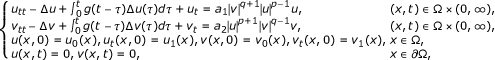(1.1)

where Ω is a bounded domain of Rn with smooth boundary ∂Ω, p > 1, q > 1 and g is a positive function. The wave equations (1.1) appear in applications in various areas of mathematical physics (see ).

If the equations in (1.1) have not the viscoelastic term, the equations are known as the wave equation. In this case, the equations have been extensively studied by many people. We observe that the wave equation subject to nonlinear boundary damping has been investigated by the authors Cavalcanti et al. [2, 3] and Vitillaro [4, 5]. It is important to mention other papers in connection with viscoelastic effects such as Aassila et al. [6, 7] and Cavalcanti et al. . Furthermore, related to blow up of the solutions of equations with nonlinear damping and source terms acting in the domain we can cite the work of Alves and Cavalcanti , Cavalcanti and Domingos Cavalcanti . As regards non-existence of a global solution, Levine  firstly showed that the solutions with negative initial energy are non-global for some abstract wave equation with linear damping. Later Levine and Serrin  studied blow-up of a class of more generalized abstract wave equations. Then Pucci and Serrin  claimed that the solution blows up in finite time with positive initial energy which is appropriately bounded. In  Levine and Todorova proved that there exist some initial data with arbitrary positive initial energy such that the corresponding solution to the wave equations blows up in finite time. Then Todorova and Vitillaro  improved the blow-up result above. However, they did not give a sufficient condition for the initial data such that the corresponding solution blows up in finite time with arbitrary positive initial energy. Recently, for problem (1.1) with g ≡ 0 and m = 1, Gazzalo and Squassina  established the condition for initial data with arbitrary positive initial energy such that the corresponding solution blows up in finite time. Zeng et al.  studied blowup of solutions for the Kirchhoff type equation with arbitrary positive initial energy.

Now we return to the problem (1.1) with g 0; in  Cavalcanti et al. first studiedand obtained an exponential decay rate of the solution under some assumption on g(s) and a(x). At this point it is important to mention some papers in connection with viscoelastic effects, among them, Alves and Cavalcanti , Aassila et al. , Cavalcanti and Oquendo  and references therein. Then Messaoudi  obtained the global existence of solutions for the viscoelastic equation, at same time he also obtained a blow-up result with negative energy. Furthermore, he improved his blow-up result in . Recently, Wang and Wang  investigated the following problemand showed the global existence of the solutions if the initial data are small enough. Moreover, they derived decay estimate for the energy functional. In  Wang established the blow-up result for the above problem when the initial energy is high.

In this paper, motivated by the work of  and employing the so called concavity argument which was first introduced by Levine (see [11, 24]), our main purpose is to establish some sufficient conditions for initial data with arbitrary positive initial energy such that the corresponding solution of (1.1) blows up in finite time. To this, we first rewrite the problem (1.1) to the following equivalent form(1.2)

where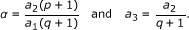We next state some assumptions on g(s) and real numbers p > 1, q > 1.

(A1) g C1([0, ∞)) is a non-negative and non-increasing function satisfying(A2) The functionis of positive type in the following sense:for all v C1([0, ∞)) and t > 0.

(A3) If n = 1, 2, then 1 < p, q < ∞. If n ≥ 3, thenRemark 1.1. It is clear that g(t) = εe -t (0 < ε < 1) satisfies the assumptions (A1) and (A2).

Based on the method of Faedo-Galerkin and Banach contraction mapping principle, the local existence and uniqueness of the problem (1.2) have been established in [8, 18, 25, 26] as follows.

Theorem 1.1. Under the assumptions (A1)-(A3), let the initial data, (u1, v1) L2(Ω) × L2(Ω). Then the problem (1.2) has a unique local solutionfor the maximum existence time T, where T (0, ∞].

Our main blow-up result for the problem (1.2) with arbitrarily positive initial energy is stated as follows.

Theorem 1.2. Under the assumptions (A1)-(A3), ifand the initial dataand (u1, v1) L2(Ω) × L2(Ω) satisfy(1.3)(1.4)(1.5)(1.6)

then the solution of the problem (1.2) blows up in finite time T < ∞, it means(1.7)

where χ is the constant of the Poincaré's inequality on Ω,, energy functional E(t) and I(u, v) are defined as(1.8)(1.9)

and.

The rest of this paper is organized as follows. In Section 2, we introduce some lemmas needed for the proof of our main results. The proof of our main results is presented in Section 3.

## 2 Preliminaries

In this section, we introduce some lemmas which play a crucial role in proof of our main result in next section.

Lemma 2.1. E(t) is a non-increasing function.

Proof. By differentiating (1.9) and using (1.2) and (A1), we get(2.1)

Thus, Lemma 2.1 follows at once. At the same time, we have the following inequality: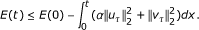(2.2)

Lemma 2.2. Assume that g(t) satisfies assumptions (A1) and (A2), H(t) is a twice continuously differentiable function and satisfies(2.3)

for every t [0, T0), and (u(x, t), v(x, t)) is the solution of the problem (1.2).

Then the function H(t) is strictly increasing on [0, T0).

Proof. Consider the following auxiliary ODE(2.4)

for every t [0, T0).

It is easy to see that the solution of (2.4) is written as follows(2.5)

for every t [0, T0).

By a direct computation, we obtainfor every t [0, T0).

Because g(t) satisfies (A2), then h'(t) ≥ 0, which implies that h(t) ≥ h(0) = H(0). Moreover, we see that H'(0) > h'(0).

Next, we show that(2.6)

Assume that (2.6) is not true, let us takeBy the continuity of the solutions for the ODES (2.3) and (2.4), we see that t0 > 0 and H' (t0) = h' (t0), and have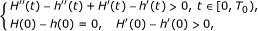which yieldsThis contradicts H'(t0) = h'(t0). Thus, we have H'(t) > h' (t) 0, which implies our desired result. The proof of Lemma 2.2 is complete.

Lemma 2.3. Suppose that, (u1, v1) L2(Ω) × L2(Ω) satisfies(2.7)

If the local solution (u(t), v(t)) of the problem (1.2) exists on [0, T) and satisfies(2.8)

thenis strictly increasing on [0, T ).

Proof. Since, and (u(t), v(t)) is the local solution of problem (1.2), by a simple computation, we have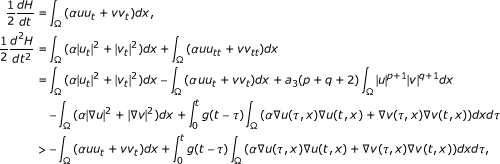which yieldsTherefore, by Lemma 2.2, the proof of Lemma 2.3 is complete.

Lemma 2.4. If, (u1, v1) L2(Ω) × L2(Ω) satisfy the assumptions in Theorem 1.2, then the solution (u(x, t), v(x, t)) of problem (1.2) satisfies(2.9)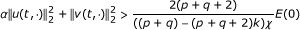(2.10)

for every t [0, T).

Proof. We will prove the lemma by a contradiction argument. First we assume that (2.9) is not true over [0, T), it means that there exists a time t1 such that(2.11)

Since I (u(t, x), v(t, x)) < 0 on [0, t1), by Lemma 2.3 we see thatis strictly increasing over [0, t1), which impliesBy the continuity ofon t, we have(2.12)

On the other hand, by (2.2) we get(2.13)

It follows from (1.9) and (2.11) that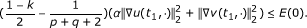(2.14)

Thus, by the Poincaré's inequality and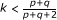, we see that(2.15)

Obviously, (2.15) contradicts to (2.12). Thus, (2.9) holds for every t [0, T).

By Lemma 2.3, it follows thatis strictly increasing on [0, T), which implies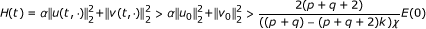for every t [0, T). The proof of Lemma 2.4 is complete.

## 3 The proof of Theorem 1.2

To prove our main result, we adopt the concavity method introduced by Levine, and define the following auxiliary function:(3.1)

where t2, t3 and a are certain positive constants determined later.

Proof of Theorem 1.2. By direct computation, we obtain(3.2)

and(3.3)

By the Young's inequality, for any ε > 0, we haveTaking, by (1.6), (2.2), (3.3), (3.4), Lemma 2.3 and the Poincaré's in-equality, we obtain(3.5)

which means that G"(t) > 0 for every t (0, T).

Since G'(0) ≥ 0 and G(0) ≥ 0, thus we obtain that G' (t) and G(t) are strictly increasing on [0, T).

It follows from (1.6) andthat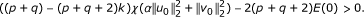Thus, we can choose a to satisfy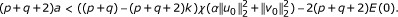SetBy (3.2) and a simple computation, for all s R, we havewhich implies that B2 - AC ≤ 0.

Since we assume that the solution (u(t, x), v(t, x)) to the problem (1.2) exists for every t [0, T), then for t [0, T), one hasandwhich yieldsLet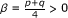. As, we see that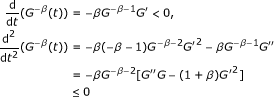(3.6)

for every t [0, T), which means that the function G is concave.

Let t2 and t3 satisfyfrom which, we deduce that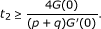Since G is a concave function and G(0) > 0, we obtain that(3.7)

thus(3.8)

Therefore, there exists a finite time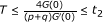such thatThe proof of Theorem 1.2 is complete.

## References

1. Fabrizio M, Morro A: Mathematical problems in linear viscoelasticity. SIAM Studies in Applied Mathematics Philadelphia 1992., 12:

2. Cavalcanti MM, Cavalcanti VND, Lasiecka I: Well-posedness and optimal decay rates for the wave equation with nonlinear boundary damping source interaction. J Diff Equ 2007, 236(2):407-459. 10.1016/j.jde.2007.02.004

3. Cavalcanti MM, Cavalcanti VND, Martinez P: Existence and decay rate estimates for the wave equation with nonlinear boundary damping and source term. J Diff Equ 2004, 203(1):119-158. 10.1016/j.jde.2004.04.011

4. Vitillaro E: A potential well theory for the wave equation with nonlinear source and boundary damping terms. Glasg Math J 2002, 44(3):375-395. 10.1017/S0017089502030045

5. Vitillaro E: Global existence for the wave equation with nonlinear boundary damping and source terms. J Diff Equ 2002, 186(1):259-298. 10.1016/S0022-0396(02)00023-2

6. Aassila M, Cavalcanti MM, Cavalcanti VND: Existence and uniform decay of the wave equation with nonlinear boundary damping and boundary memory source term. Calc Var Partial Diff Equ 2002, 15(2):155-180. 10.1007/s005260100096

7. Aassila M, Cavalcanti MM, Soriano JA: Asymptotic stability and energy decay rates for solutions of the wave equation with memory in a star-shaped domain. SIAM J Control Optim 2000, 38(5):1581-1602. 10.1137/S0363012998344981

8. Cavalcanti MM, Cavalcanti VND, Soriano JA: Existence and uniform decay rate for viscoelastic problems with nonlinear boundary damping. Diff Integ Equ 2001, 14: 85-116.

9. Alves CO, Cavalcanti MM: On existence, uniform decay rates and blow up for solutions of the 2-D wave equation with exponential source. Calc Var Partial Diff Equ 2009, 34(3):377-411. 10.1007/s00526-008-0188-z

10. Cavalcanti MM, Cavalcanti VND: Existence and asymptotic stability for evolution problems on manifolds with damping and source terms. J Math Anal Appl 2004, 291(1):109-127. 10.1016/j.jmaa.2003.10.020

11. Levine HA: Some additional remarks on the nonexistence of global solutions to nonlinear wave equations. SIAM J Math Anal 1974, 5: 138-146. 10.1137/0505015

12. Levine HA, Serrin J: Global nonexistence theorems for quasilinear evolution equation with dissipation. Arch Ration Mech Anal 1997, 137: 341-361. 10.1007/s002050050032

13. Pucci P, Serrin J: Global nonexistence for abstract evolution equation with positive initial energy. J Diff Equ 1998, 150: 203-214. 10.1006/jdeq.1998.3477

14. Levine HA, Todorova G: Blow up of solutions of the Cauchy problem for a wave equation with nonlinear damping and source terms and positive initial energy. Proc Am Math Soc 2001, 129: 793-805. 10.1090/S0002-9939-00-05743-9

15. Todorova G, Vitillaro E: Blow-up for nonlinear dissipative wave equations in Rn . J Math Anal Appl 2005, 303: 242-257. 10.1016/j.jmaa.2004.08.039

16. Gazzola F, Squassina M: Global solutions and finite time blow up for damed semilinear wave equations. Ann Inst H Poincare Anal NonLineaire 2006, 23(2):185-207. 10.1016/j.anihpc.2005.02.007

17. Zeng R, Mu CL, Zhou SM: A blow up result for Kirchhoff type equations with high energy. Math Methods Appl Sci 2011, 34(4):479-486.

18. Cavalcanti MM, Cavalcanti VND, Soriano JA: Exponential decay for the solution of the semilinear viscoelastic wave equations with localized damping. Electron J Diff Equ 2002, 44: 1-14.

19. Cavalcanti MM, Oquendo HP: Frictional versus viscoelastic damping in a semilinear wave equation. SIAM J Control Optim 2003, 42(4):1310-1324. 10.1137/S0363012902408010

20. Messaoudi SA: Blow up and global existence in a nonlinear viscoelastic wave equation. Math Nachr 2003, 260: 58-66. 10.1002/mana.200310104

21. Messaoudi SA: Blow up of positive-initial-energy solutions of a nonlinear viscoelastic hyperbolic equation. J Math Anal Appl 2006, 320: 902-915. 10.1016/j.jmaa.2005.07.022

22. Wang YJ, Wang YF: Exponential energy decay of solutions of viscoelastic wave equations. J Math Anal Appl 2008, 347: 18-25. 10.1016/j.jmaa.2008.05.098

23. Wang YJ: A global nonexistence theorem for viscoelastic equations with arbitrary positive initial energy. Appl Math Lett 2009, 22: 1394-1400. 10.1016/j.aml.2009.01.052

24. Levine HA: Instability and nonexistence of global solutions of nonlinear wave equation of the form Pu tt = Δ u + F ( u ). Trans Am Math Soc 1974, 192: 1-21.

25. Cavalcanti MM, Cavalcanti VND, Ferreira J: Existence and uniform decay for nonlinear viscoelastic equation with strong damping. Math Meth Appl Sci 2001, 24: 1043-1053. 10.1002/mma.250

26. Georgiev V, Todorova G: Existence of a solution of the wave equation with nonlinear damping and source term. J Diff Equ 1994, 109: 295-308. 10.1006/jdeq.1994.1051

## Acknowledgements

This work is supported in part by NSF of PR China (11071266) and in part by Natural Science Foundation Project of CQ CSTC (2010BB9218).

## Author information

Authors

### Corresponding author

Correspondence to Jie Ma.

### Competing interests

The authors declare that they have no competing interests.

### Authors' contributions

MJ and CL carried out all studies in the paper. ZR participated in the design of the study in the paper.

## Rights and permissions

Reprints and Permissions

Ma, J., Mu, C. & Zeng, R. A blow up result for viscoelastic equations with arbitrary positive initial energy. Bound Value Probl 2011, 6 (2011). https://doi.org/10.1186/1687-2770-2011-6